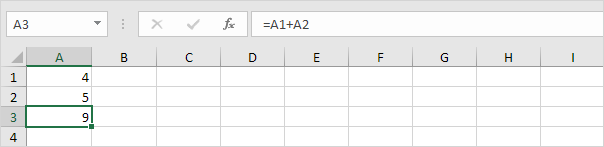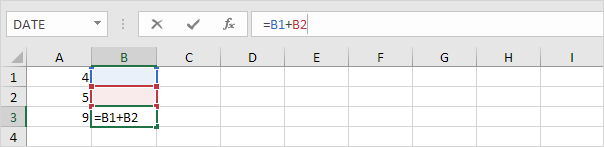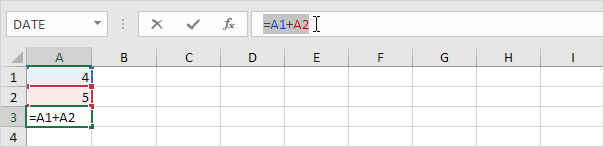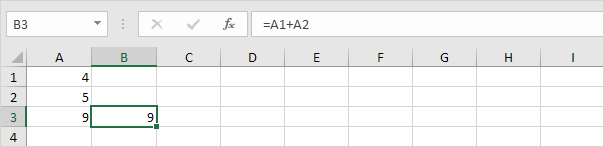## Excel Office

Excel How Tos, Tutorials, Tips & Tricks, Shortcuts

# How to Copy, Paste and Retain Exact Copied Formula in another Cell in Excel

When you copy a formula, Excel automatically adjusts the cell references for each new cell the formula is copied to.

For example, cell A3 below contains a formula which adds the value of cell A2 to the value of cell A1.

Worked Example:   How to get address of named range in ExcelWhen you copy this formula to cell B3 (select cell A3, press CTRL + c, select cell B3, press CTRL + v), the formula will automatically reference the values in column B.If you don’t want this but instead want to copy the exact formula (without changing the cell references), execute the following easy steps.

Worked Example:   How to get original price from percentage discount in Excel

1. Click in the formula bar and select the formula.2. Press CTRL + c, and press Enter.

3. Select cell B3 and click in the formula bar again.

Worked Example:   Superscript and Subscript in Excel

4. Press CTRL + v, and press Enter.

Result:Both cell A3 and cell B3 contain the exact same formula now.

You can also visit: Cell References: Relative, Absolute and Mixed Referencing Examples. for more illustrations.